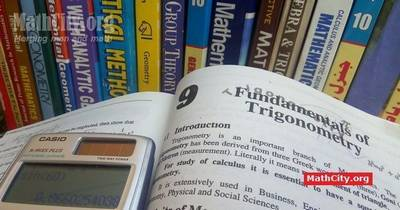# Chapter 09: Fundamentals of TrigonometryNotes (Solutions) of Chapter 09: Fundamentals of Trigonometry, Text Book of Algebra and Trigonometry Class XI (Mathematics FSc Part 1 or HSSC-I), Punjab Text Book Board, Lahore. This chapter has four exercise and solutions of those exercises are given below which can be downloaded in PDF format or can be viewed online.

• Introduction
• Units of Measures of Angles
• Sexagesimal System
• Conversion from $D^\circ M'S''$ to the decimal form and vice versa
• Relation between the Length of an arc of a Circle and the Circular measure of its Central angle
• Conversion of Radian into Degree and vice versa
• Exercise 9.1
• General Angle (Coterminal Angle)
• Angle in the Standard Position
• Trigonometric Function
• Trigonometric Function of any Angle
• Fundamental Identities
• Signs of the Trigonometric Function
• Exercise 9.2
• The Values of Trigonometric Functions of acute angles $45^\circ , 30^\circ , 60^\circ$
• The Values of Trigonometric Functions of acute angles $0^\circ , 90^\circ , 180^\circ , 270^\circ , 360^\circ$
• Exercise 9.3
• Domains of Trigonometric Functions and of Fundamental Identities
• Exercise 9.4

#### Notes by Akhtar Abbas

The following notes are provided by Mr. Akhtar Abbas. A video explanation of the solutions is available at his YouTube channel Suppose Math.

• Exercise 9.1 | Download PDF• Exercise 9.2 | Download PDF• Exercise 9.3 | Download PDF• Exercise 9.4 | Download PDFThe following short questions was send by Mr. Akhtar Abbas.

• fsc/fsc_part_1_solutions/ch09